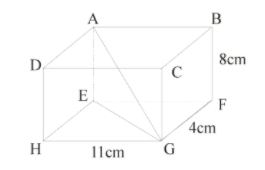If is a cuboid, find length of .

# Ifis a cuboid, find length of.1. A2. B3. C4. DFill Out the Form for Expert Academic Guidance!l

+91

Live ClassesBooksTest SeriesSelf Learning

Verify OTP Code (required)

### Solution:

Concept- Cuboids have six faces, eight vertices, and twelve edges and seem to be three-dimensional shapes. A cuboid's length, width, and height are all different.
In,
By Pythagoras theorem,In,
By Pythagoras theorem,Hence, the correct answer is option 3.

## Related content

 Area of Square Area of Isosceles Triangle Pythagoras Theorem Triangle Formula Perimeter of Triangle Formula Area Formulae Volume of Cone Formula Matrices and Determinants_mathematics Critical Points Solved Examples Type of relations_mathematics+91

Live ClassesBooksTest SeriesSelf Learning

Verify OTP Code (required)